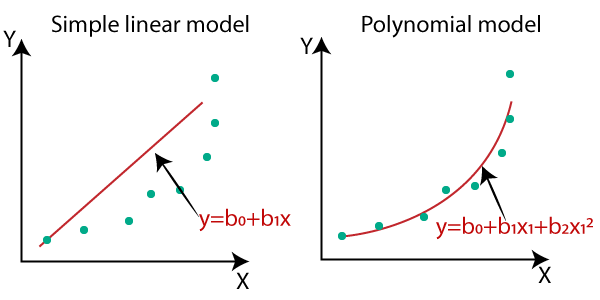# Why Polynomial regression required?

## Need for Polynomial Regression:

The need of Polynomial Regression in ML can be understood in the below points:

• If we apply a linear model on a linear dataset , then it provides us a good result as we have seen in Simple Linear Regression, but if we apply the same model without any modification on a non-linear dataset , then it will produce a drastic output. Due to which loss function will increase, the error rate will be high, and accuracy will be decreased.
• So for such cases, where data points are arranged in a non-linear fashion, we need the Polynomial Regression model . We can understand it in a better way using the below comparison diagram of the linear dataset and non-linear dataset.• In the above image, we have taken a dataset which is arranged non-linearly. So if we try to cover it with a linear model, then we can clearly see that it hardly covers any data point. On the other hand, a curve is suitable to cover most of the data points, which is of the Polynomial model.
• Hence, if the datasets are arranged in a non-linear fashion, then we should use the Polynomial Regression model instead of Simple Linear Regression.